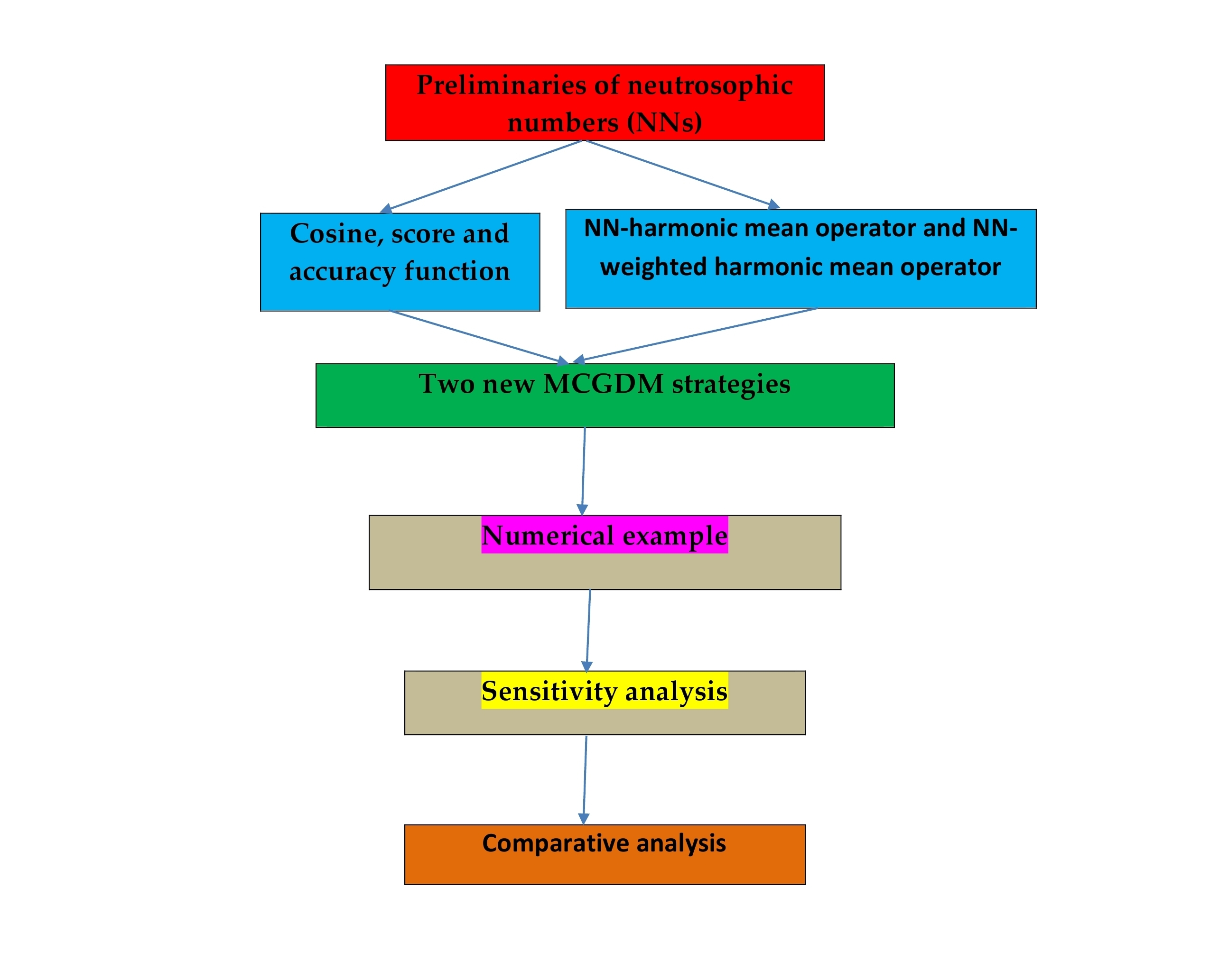Preprint Article Version 1 Preserved in Portico This version is not peer-reviewed

# NN-Harmonic Mean Aggregation Operators Based MCGDM Strategy in Neutrosophic Number Environment

Version 1 : Received: 19 November 2017 / Approved: 20 November 2017 / Online: 20 November 2017 (09:53:31 CET)

A peer-reviewed article of this Preprint also exists.

Mondal, K.; Pramanik, S.; Giri, B.C.; Smarandache, F. NN-Harmonic Mean Aggregation Operators-Based MCGDM Strategy in a Neutrosophic Number Environment. Axioms 2018, 7, 12. Mondal, K.; Pramanik, S.; Giri, B.C.; Smarandache, F. NN-Harmonic Mean Aggregation Operators-Based MCGDM Strategy in a Neutrosophic Number Environment. Axioms 2018, 7, 12.

## Abstract

The concept of neutrosophic number is a significant mathematical tool to deal with real scientific problems because it can tackle indeterminate and incomplete information which exists generally in real problems. In this article, we use neutrosophic numbers (a + bI), where a and bI denote determinate component and indeterminate component respectively. We explore the situations in which the input information is needed to express in terms of neutrosophic numbers. We define score functions and accuracy functions for ranking neutrosophic numbers. We then define a cosine function to determine unknown criteria weights. We define neutrosophic number harmonic mean operators and proved their basic properties. Then, we develop two novel MCGDM strategies using the proposed aggregation operators. We solve a numerical example to demonstrate the feasibility and effectiveness of the proposed two strategies. Sensitivity analysis with variation of “I” on neutrosophic numbers is performed to demonstrate how the preference ranking order of alternatives is sensitive to the change of “I”. The efficiency of the developed strategies is ascertained by comparing the obtained results from the proposed strategies with the existing strategies in the literature.## Keywords

neutrosophic number; neutrosophic number harmonic mean operator (NNHMO); neutrosophic number weighted harmonic mean operator (NNWHMO); cosine function, score function; multi criteria group decision making

## Subject

Computer Science and Mathematics, Applied Mathematics